# C++ Unary Operators

The unary operators take a single argument in C++ language. Some of the operators you have already seen in previous articles are unary operators.

Here is the list of unary operators.

Dereference Operator (*)

When a C++ pointer type is declared, and it is pointing to a variable. The dereference operator helps to access the content of a pointer type. We will learn more about while discussing pointers.

It resembles the bitwise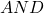, but when used as a unary operator it returns the address of the variable.

int A = &B;

The variable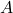will store address of the variable B, not its value.

Minus Sign (-)

Minus is an arithmetic operator, but as a unary operator, it is used to denote a negative value. It is most commonly used the unary operator in C++ programs.

Negation Operator(!)

The negation is a logical operator which negates the value of a boolean variable. If the value is(true) then, it becomes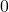(false). If the value is(false) then, it becomes(true).

Bitwise Complement (~)

The bitwise complement modifies the binary value of a variable to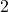‘s complement binary value. In other words, you get a negative value for a positive number plus.

\begin{aligned}p = 45  \hspace{5px} becomes \hspace{5px} -46 \hspace{5px} after \hspace{5px} \sim p.\end{aligned}

Increment Operator (++)

The increment operator is used in loop structures. You will learn about loop structures later in this C++ tutorial. The increment operator increments an associated variable by.

i = 12;
i++; // same as saying i = i + 1;

The value ofafter increment is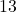.

Decrement Operator (–)

The decrement operator decreases the associated variable value by.

i = 34;
i--;

The value will be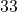after decrement.

Type Operator

The type conversion operator is used to modify the data type of a variable by force. The initial data type is changed to what specified in the type operator. It is also known as cast operator.

int A;
A = 10;
(float) A;

This will change the variableinto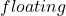type from an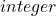.

Sizeof Operator( sizeof)

The sizeof operator is a special operator that returns the size of the data type in C++ program.

int A;
sizeof(A);

This will return the size of integer data type which is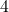bytes.